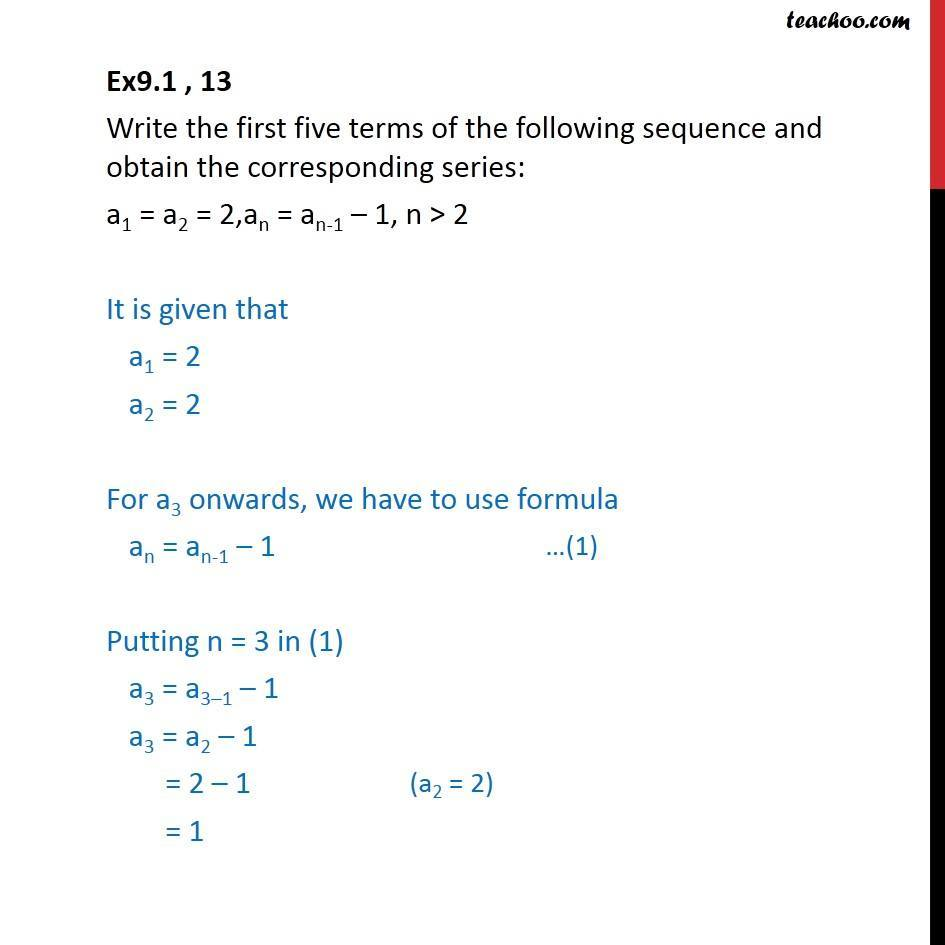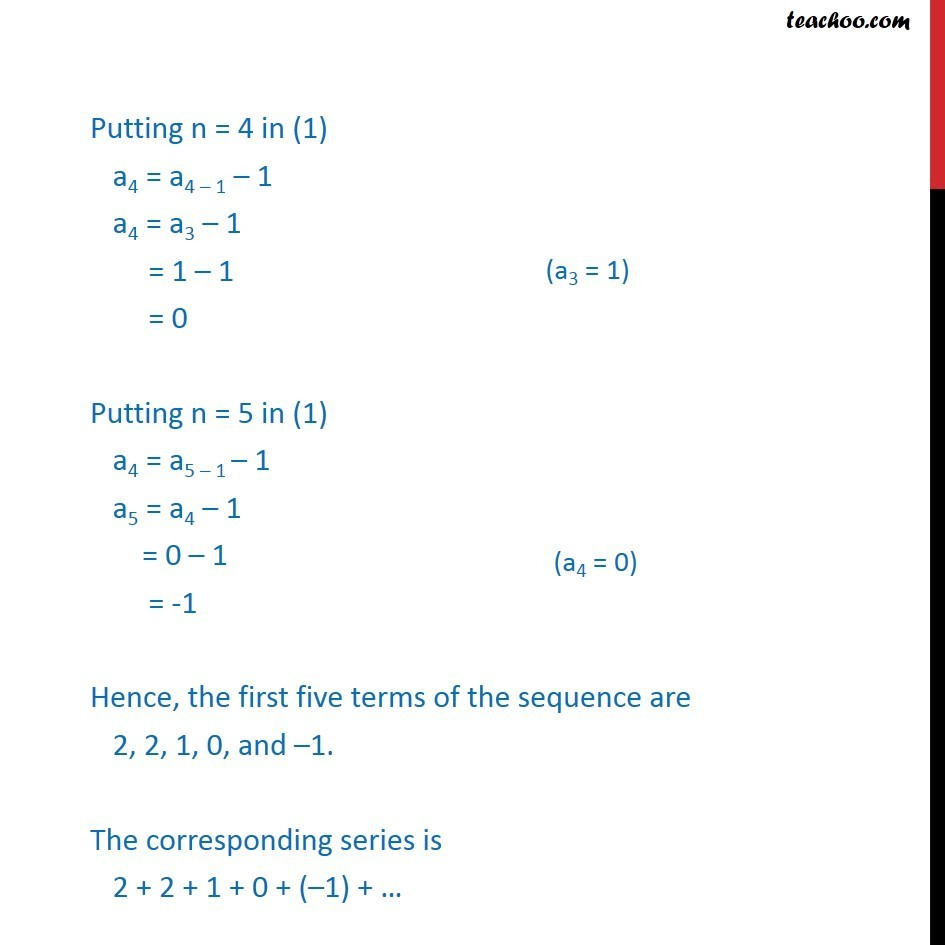Ex 8.1

Chapter 8 Class 11 Sequences and Series
Serial order wiseLearn in your speed, with individual attention - Teachoo Maths 1-on-1 Class

### Transcript

Ex9.1 , 13 Write the first five terms of the following sequence and obtain the corresponding series: a1 = a2 = 2,an = an-1 – 1, n > 2 It is given that a1 = 2 a2 = 2 For a3 onwards, we have to use formula an = an-1 – 1 Putting n = 3 in (1) a3 = a3–1 – 1 a3 = a2 – 1 = 2 – 1 = 1 Putting n = 4 in (1) a4 = a4 – 1 – 1 a4 = a3 – 1 = 1 – 1 = 0 Putting n = 5 in (1) a4 = a5 – 1 – 1 a5 = a4 – 1 = 0 – 1 = -1 Hence, the first five terms of the sequence are 2, 2, 1, 0, and –1. The corresponding series is 2 + 2 + 1 + 0 + (–1) + …